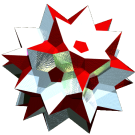Acronym tikit Name triakis tetrahedron,apiculated tetrahedron© Inradius 5/sqrt(88) = 0.533002 Vertex figure [t6], [T3] Dihedral angles at long edge:   arccos(-7/11) = 129.521196° at short edge:   arccos(-7/11) = 129.521196° Dual tut ExternallinksNote that this polyhedron also is the hull of a complex of 5 tets of the small edge size. The vertices of the central tet of that complex thus define the shallow tips. Its edges are of the same size as the shorter edges of the hull, but would be completely internal.

The triangles {(t,t,T)} have vertex angles t = arccos(5/6) = 33.557310° resp. T = arccos(-7/18) = 112.885380°.

Edge sizes used here are tT = x = 1 (short) resp. tt = a = 5/3 = 1.666667 (long).

Incidence matrix according to Dynkin symbol

m3m3o =
ao3oo3ox&#zx   → height = 0
a = 5/3 = 1.666667

o.3o.3o.    | 4 * | 3  3 |  6  [t6]
.o3.o3.o    | * 4 | 0  3 |  3  [T3]
------------+-----+------+---
a. .. ..    | 2 0 | 6  * |  2  a
oo3oo3oo&#x | 1 1 | * 12 |  2  x
------------+-----+------+---
ao .. ..&#x | 2 1 | 1  2 | 12  {(t,t,T)}